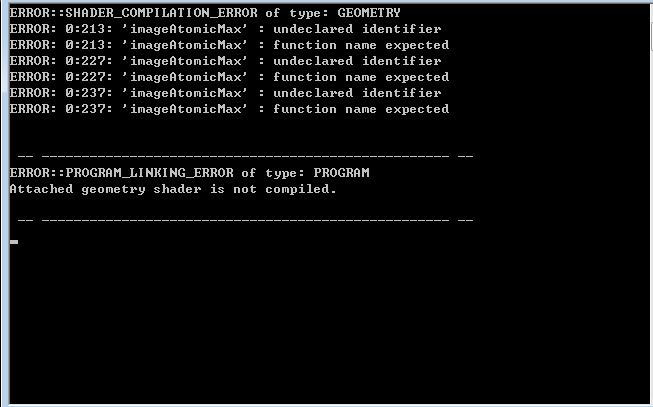# Cannot use Image variables and Shader Storage Blocks in geometry shader？

Hello！
I’m confused about the use of Image variables and Shader Storage Blocks in geometry shader.
I defined a Image variables and a Shader Storage Block in geometry shader，and used some atomic functions such as “ imageAtomicMax” and “imageAtomicAdd”. When I compile my program, it prompts for a geometry shader compilation error with following tips：“imageAtomicMax” undeclared identifier， “imageAtomicMax” function name ecpected.
So is the Image variables and Shader Storage Blocks can not be used in geometry shader? however, in the OpenGL SuperBible book, it makes it clear that any shader stage can write data into images, not just fragment shaders. Can anyone give me a specific explanation? Sincerely hope to get your answer, thank you very much！

While it is possible for an implementation to state that the number of Geometry Shader image variables is zero (that is, the standard only requires implementations let you do image load/store and SSBOs from the fragment shader), apparently zero implementations of desktop OpenGL actually forbid the use of image load/store from non-fragment shader stages.

So assuming that you’re not using some odd-ball implementation (or perhaps OpenGL ES, where things may be less clear-cut), then the problem is likely in your `#version` declaration. Image load/store is part of GLSL 4.20, and SSBOs are part of GLSL 4.30. So you have to use the appropriate `#version` declaration to gain access to them.

That being said, it would be best to post the entire text of the shader, along with the exact text of the error message.

Thanks very much for your kind reply！ In my shader program,i did using GLSL 4.30, but the error is still extence.My geometey shader and the corresponding Compile error are show as follows：

``````#version 430 core
layout (points) in;
layout (points,max_vertices = 1) out;

layout (binding=0,r32ui)uniform uimageBuffer colliValueImage;

in vec4 word_space_pos[];
in int instancedID[];

out int collision_index;
out float collisionValue;

uniform vec3 P1;
uniform vec3 P2;
uniform vec3 P9;
uniform vec3 P10;
uniform vec3 P11;
uniform vec3 P4;
uniform vec3 P5;
uniform vec3 P6;

uniform vec3 origin;

uniform mat4 worldToTCP;

bool PointInCircular(vec3 detectionPnt, vec3 centerPnt, float radius, out float collDimension)
{
vec3 pointToCenter = detectionPnt - centerPnt;
float alength = length(pointToCenter);
bool InCircular;
{
collDimension = radius -alength;
InCircular = true;
}
else
{
collDimension = 0;
InCircular = false;
}
return InCircular;//点到圆心的距离小于端面圆半径时，在园内
}

float CalculatePointToLineDistance(vec3 LinePA, vec3 LinePB, vec3 P)
{
vec3 vAB = LinePB - LinePA;
vec3 vAP = P - LinePA;
float lengthAP = length(vAP);
vAB = normalize(vAB);
float a = dot(vAP,vAB);
float b = lengthAP * lengthAP - a * a;
return sqrt(b);
}

bool SameSide(vec3 A, vec3 B, vec3 C, vec3 P)
{
vec3 AB = B - A ;
vec3 AC = C - A ;
vec3 AP = P - A ;

vec3 v1 = cross(AB,AC);
vec3 v2 = cross(AB,AP);

// v1 and v2 should point to the same direction
return dot(v1,v2) >= 0 ;
}

bool PointinTriangle2(vec3 A, vec3 B, vec3 C, vec3 P, out float collDimension)
{
bool inTri = SameSide(A, B, C, P) && SameSide(B, C, A, P) && SameSide(C, A, B, P);
if(inTri)
{
collDimension = CalculatePointToLineDistance(A,B,P);
}
return inTri;
}

bool PointInRectangle(vec3 rect1, vec3 rect2, vec3 P)
{
vec3 pointA = rect1;
vec3 pointB = rect2 ;
vec3 pointC = rect2 + 30.0 * vec3(1,0,0);
vec3 pointD = rect1 + 30.0 * vec3(1,0,0);
if(pointA.x <= P.x && P.x <=pointD.x && pointB.y < P.y && P.y <= pointA.y)
{
return true;
}
else
return false;
}

bool PointInRectangle4(vec3 rect1, vec3 rect2,vec3 rect3, vec3 rect4,vec3 P)
{
if(rect2.z <= P.z && P.z <=rect1.z && rect3.y <= P.y && P.y <= rect2.y)
{
return true;
}
else
return false;
}

bool PointinTriangle(vec3 A, vec3 B, vec3 C, vec3 P, inout float collDimension)
{
vec3 v0 = C - A ;
vec3 v1 = B - A ;
vec3 v2 = P - A ;

float dot00 = dot(v0,v0);
float dot01 = dot(v0,v1);
float dot02 = dot(v0,v2);
float dot11 = dot(v1,v1);
float dot12 = dot(v1,v2); ;

float inverDeno = 1 / (dot00 * dot11 - dot01 * dot01) ;

float u = (dot11 * dot02 - dot01 * dot12) * inverDeno ;
if (u < 0 || u > 1) // if u out of range, return directly
{
collDimension = 0;
return false ;
}

float v = (dot00 * dot12 - dot01 * dot02) * inverDeno ;
if (v < 0 || v > 1) // if v out of range, return directly
{
collDimension = 0;
return false ;
}
bool inTriangle;
if(u + v <= 1)
{
collDimension = CalculatePointToLineDistance(A,B,P);
inTriangle = true;

}
else
{
collDimension = 0;
inTriangle = false;
}
return inTriangle ;
}

void main()
{
vec3 projCoords = gl_in.gl_Position.xyz / gl_in.gl_Position.w; //透视除法，转换为归一化标准坐标
if(projCoords.x >= -1 && projCoords.x<= 1 && projCoords.y >= -1 && projCoords.y<= 1 && projCoords.z >= -1 && projCoords.z<= 1)
{
vec3 tcp_space_position = vec3(worldToTCP * word_space_pos);
if(PointInRectangle4(P1,P2,origin,P9,tcp_space_position))//干涉点位于矩形P1P2P7P9内
{
collision_index = 1;
collisionValue = CalculatePointToLineDistance(vec3(0,P1.yz),vec3(0,P2.yz),vec3(0,tcp_space_position.yz));
uint uiColliValue = uint(collisionValue * 1000);
imageAtomicMax(colliValueImage,instancedID,uiColliValue);
gl_Position = gl_in.gl_Position;
EmitVertex();
}
else if(PointInRectangle4(P2,P10,P11,P4,tcp_space_position))//干涉点位于矩形P2P10P11P4内
{
float collValue = 0;
bool isCollosion = PointInCircular(vec3(0,tcp_space_position.yz),vec3(0,origin.yz),wheelRadius, collValue);
if(isCollosion)
{
collision_index = 1;
collisionValue = collValue;
uint uiColliValue = uint(collisionValue * 1000);

imageAtomicMax(colliValueImage,instancedID,uiColliValue);
gl_Position = gl_in.gl_Position;
EmitVertex();
}
}
else if(PointInRectangle4(P6,origin,P4,P5,tcp_space_position))
{
collision_index = 1;
collisionValue = CalculatePointToLineDistance(vec3(0,P4.yz),vec3(0,P5.yz),vec3(0,tcp_space_position.yz));
uint uiColliValue = uint(collisionValue * 1000);
imageAtomicMax(colliValueImage,instancedID,uiColliValue);
gl_Position = gl_in.gl_Position;
EmitVertex();
}
}
EndPrimitive();
}
``````Here (AMD Radeon HD 7800), I get no compilation errors for that shader, only a couple of warnings that integer inputs/outputs should have the `flat` qualifier.

Does your hardware/driver support 4.3?

Also, that shader doesn’t use SSBOs and should work with 4.2.